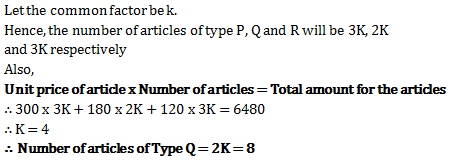# Ratio and Proportion - Quantitative Aptitude (MCQ) questions for Q. 29266

Q.  Price of each article of type P, Q, and R is Rs. 300, Rs. 180 and Rs. 120 respectively. Suresh buys articles of each type in the ratio 3:2:3 in Rs. 6480. How many articles of type Q did he purchase?
- Published on 11 Apr 17

a. 8
b. 14
c. 20
d. None of the above